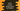# How to convert a string to float and double in Swift## How to convert a string to float and double in Swift:

A string can hold a valid float or double value and if we want to convert that value to flooat or double, we can do that easily in swift. String holding doubles are longer than string holding float. Double is for 64 bit floating point number and Float is for 32 bit numbers.

In this post, we will learn how to convert a string to float and double in Swift.

### Convert string to float:

Swift strings doesn’t have any method to convert to float. But, we can use NSString for that. NSString has floatValue property that returns the floating point value of a string. Let’s try this method with different values:

``````import Foundation

let givenValues = ["1.223", "1.23xy", ".3", "0.3", "0.3e", "abcd"]

for item in givenValues{
print("\(item) => \((item as NSString).floatValue)")
}``````

This program will print the below output:

``````1.223 => 1.223
1.23xy => 1.23
.3 => 0.3
0.3 => 0.3
0.3e => 0.3
abcd => 0.0``````

### Using Float() constructor:

We can pass one string value to the Float constructor. It returns one optional value. For a string that can’t be converted, it returns nil. Let’s take a look at the below program:

``````import Foundation

let givenValues = ["1.223", "1.23xy", ".3", "0.3", "0.3e", "abcd"]

for item in givenValues{
print("\(item) => \(Float(item))")
}``````

It will print:

``````1.223 => Optional(1.223)
1.23xy => nil
.3 => Optional(0.3)
0.3 => Optional(0.3)
0.3e => nil
abcd => nil``````

As you can see, the first method returns 0.0, but it returns nil. We need to use one if let block to safely unwrap the value.

``````import Foundation

let givenValues = ["1.223", "1.23xy", ".3", "0.3", "0.3e", "abcd"]

for item in givenValues{
if let floatValue = Float(item) {
print("\(item) => \(floatValue)")
}else{
print("Can't convert \(item)")
}
}``````

It will print:

``````1.223 => 1.223
Can't convert 1.23xy
.3 => 0.3
0.3 => 0.3
Can't convert 0.3e
Can't convert abcd``````

### Convert string to double:

We can convert a string to double using similar approaches. We can read the doubleValue on NSString.

``````import Foundation

let givenValues = ["1.22377673636373", "1.2332323223xy", ".321212121213", "0.31111", "0.3e", "abcd"]

for item in givenValues{
print("\(item) => \((item as NSString).doubleValue)")
}``````

It prints the below output:

``````1.22377673636373 => 1.22377673636373
1.2332323223xy => 1.2332323223
.321212121213 => 0.321212121213
0.31111 => 0.31111
0.3e => 0.3
abcd => 0.0``````

Similarly, we can use Double constructor :

``````import Foundation

let givenValues = ["1.22377673636373", "1.2332323223xy", ".321212121213", "0.31111", "0.3e", "abcd"]

for item in givenValues{
print("\(item) => \(Double(item))")
}``````

It will print:

``````1.22377673636373 => Optional(1.22377673636373)
1.2332323223xy => nil
.321212121213 => Optional(0.321212121213)
0.31111 => Optional(0.31111)
0.3e => nil
abcd => nil``````